# Methods and formulas for 2-Sample Poisson Rate

Select the method or formula of your choice.

## Statistics

Minitab generates the following descriptive statistics from your sample. Minitab displays a mean only if you change "length" from its default value of 1.
TermDescription
rate of occurrence for sample i
TermDescription
mean number of occurrences in sample i

## Hypothesis test for a difference in rates for the normal approximation

### Formula

The normal approximation test is based on the following Z-statistic, which is approximately distributed as a standard normal distribution under the null hypothesis:

Minitab uses the following p-value equations for the respective alternative hypotheses:

### Notation

TermDescription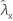observed value of rate for sample X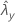observed value of rate for sample Y
ζ true value of the difference between the population rates of two samples
ζ0 hypothesized value of the difference between the population rates of two samples
m sample size of sample X
n sample size of sample Y
tx length of sample X
ty length of sample Y

## Hypothesis test for a difference in rates for the exact method

### Formula

When the hypothesized difference equals 0, Minitab uses an exact procedure to test the following null hypothesis:

H0: ζ = λxλy = 0, or H0: λx = λy

The exact procedure is based on the following fact, assuming the null hypothesis is true:

S | W ~ Binomial(w, p)

where:

W = S + U

Minitab uses the following p-value equations for the respective alternative hypotheses:
• H1: ζ > 0: p-value = P(S s | w = s + u, p = p0)

• H1: ζ < 0: p-value = P(S s | w = s + u, p = p0)

• H1: ζ ≠ 0:
• if P(S s | w = s + u, p = p0) ≤ 0.5 or P(S s | w = s + u, p = p0) ≤ 0.5

then the p-value = 2 × min {P(S s | w = s + u, p = p0), P(S s | w = s + u, p = p0)}

• otherwise, p-value = 1.0

where:

### Notation

TermDescription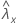observed value of the rate for sample X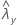observed value of the rate for sample Y
λxtrue value of the rate for population X
λytrue value of rate for population Y
ζtrue value of the difference between the population rates of two samples
txlength of sample X
tylength of sample Y
msample size of sample X
nsample size of sample Y

## Hypothesis test for a difference in rates with the pooled rate method

When you test a zero difference with the following null hypothesis, you have the option to use a pooled rate for both samples:

### Formula

The pooled-rate procedure is based on the following Z-statistic, which is approximately distributed as a standard normal distribution under the following null hypothesis:

where:

Minitab uses the following p-value equations for the respective alternative hypotheses:

### Notation

TermDescriptionobserved value of the rate for sample Xobserved value of the rate for sample Y
λxtrue value of the rate for population X
λytrue value of the rate for population Y
ζtrue value of the difference between the population rates of two samples
msample size of sample X
nsample size of sample Y
txlength of sample X
tylength of sample Y

## Hypothesis test for a difference in means for the normal approximation method

### Formula

The normal approximation test is based on the following Z-statistic, which is approximately distributed as a standard normal distribution under the null hypothesis.

Minitab uses the following p-value equations for the respective alternative hypotheses:

### Notation

TermDescription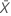observed value of the mean number of occurrences in sample X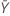observed value of the mean number of occurrences in sample Y
δ true value of the difference between the population means of two sample
δ 0 hypothesized value of the difference between the population means of two samples
m sample size of sample X
n sample size of sample Y

## Hypothesis test for a difference in means for the exact method

### Formula

When the hypothesized difference equals 0, Minitab uses an exact procedure. The exact procedure uses the following null hypothesis:

The exact procedure is based on the following fact, assuming the null hypothesis is true:

S | W ~ Binomial(w, p)

where:

W = S + U

Minitab uses the following p-value equations for the respective alternative hypotheses:

H1: δ > 0: p-value = P(S s | w = s + u, δ = 0)

H1: δ < 0: p-value = P(S s | w = s + u, δ = 0)

H1: δ ≠ 0:
• if P(Ss|w = s + u, δ = 0) ≤ 0.5

or P(Ss|w = s + u, δ = 0) ≤ 0.5

then: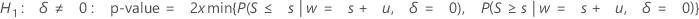• otherwise, p-value = 1.0

A two-tailed test is not an equal-tailed test unless m = n.

### Notation

TermDescription
μx true value of the mean number of occurrences in population X
μytrue value of the mean number of occurrences in population Y
δtrue value of the difference between the population means of two samples
msample size of sample X
nsample size of sample Y

## Hypothesis test for a difference in means for the pooled mean method

### Formula

When you test a zero difference with the following null hypothesis, you have the option to use a pooled rate for both samples:

The pooled-mean procedure is based on the following Z-value, which is approximately distributed as a standard normal distribution under the following null hypothesis:

where:

Minitab uses the following p-value equations for the respective alternative hypotheses:

### Notation

TermDescriptionobserved value of the mean number of occurrences in sample Xobserved value of the mean number of occurrences in sample Y
µxtrue value of the mean number of occurrences in population X
µytrue value of the mean number of occurrences in population Y
δtrue value of the difference between the population means of two samples
msample size of sample X
nsample size of sample Y

## Confidence interval for the difference in rates

### Formula

A 100(1 – α)% confidence interval for the difference between two population Poisson rates is given by:

### Notation

TermDescriptionobserved value of rate for sample Xobserved value of rate for sample Y
ζtrue value of the difference between the population rates of two samples
zxupper x percentile point of the standard normal distribution, where 0 < x < 1
msample size of sample X
nsample size of sample Y
txlength of sample X
tylength of sample Y

## Confidence bounds for the difference in rates

### Formula

When you specify a "greater than" test, a 100(1 – α)% lower confidence bound for the difference between two population Poisson rates is given by:

When you specify a "less than" test, a 100(1 – α)% upper confidence bound for the difference between two population Poisson rates is given by:

### Notation

TermDescriptionobserved value of rate for sample Xobserved value of rate for sample Y
ζtrue value of the difference between the population rates of two samples
zxthe upper x percentile point on the standard normal distribution, where 0 < x < 1
msample size of sample X
nSample size of sample Y
txlength of sample X
tylength of sample Y

## Confidence interval for the difference in means

### Formula

A 100(1 – α)% confidence interval for the difference between two population Poisson means is given by:

### Notation

TermDescriptionobserved value of the mean number of occurrences in sample Xobserved value of the mean number of occurrences in sample Y
δtrue value of the difference between the population means of two samples
zxupper x percentile point on the standard normal distribution, where 0 < x < 1
msample size of sample X
nsample size of sample Y

## Confidence bounds for the difference in means

### Formula

When you specify a "greater than" test, a 100(1 – α)% lower confidence bound for the difference between two population Poisson means is given by:

When you specify a "less than" test, a 100(1 – α)% upper confidence bound for the difference between two population Poisson means is given by:

### Notation

TermDescriptionobserved value of the mean number of occurrences in sample Xobserved value of the mean number of occurrences in sample Y
δtrue value of the difference between the population means of two samples
zxupper x percentile point on the standard normal distribution, where 0 < x < 1
msample size of sample X
nsample size of sample Y
By using this site you agree to the use of cookies for analytics and personalized content.  Read our policy